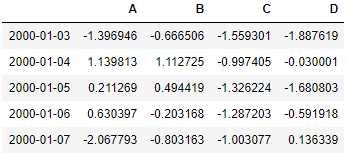# How to create dataframe for testing?

Did you ever wanted to create dataframes for testing and find it hard to fill the dataframe with dummy values then DO NOT Worry there are functions that are not mentioned in the official document but available in pandas util modules which can be used to create the dataframes and we will explore those methods in this post.

Here are the functions that you can directly use to create the test dataframes

## Random Dataframe

it creates a dummy dataframe with random real number of 4 columns A,B,C,D

The index is a group of alpha-numeric character

``````from pandas import util
df= util.testing.makeDataFrame()
``````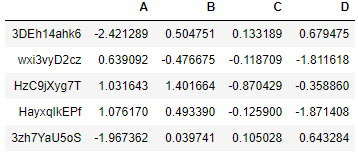## Missing Dataframe

It creates dataframe with missing values in it. You can see those NaN values in Column A

``````df= util.testing.makeMissingDataframe()
``````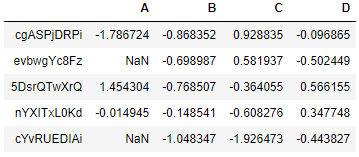## Time Dataframe

It creates the time-series dataframe

``````df= util.testing.makeTimeDataFrame()
``````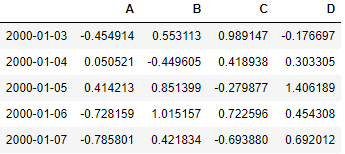## Mixed dataframe

It creates a mixed dataframe containing Categorical, date-time and Continuous columns

``````df= util.testing.makeMixedDataFrame()
``````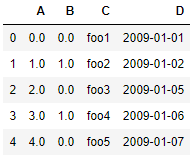## Period Frame

It creates time-series dataframe with periodical data

``````df=util.testing.makePeriodFrame()